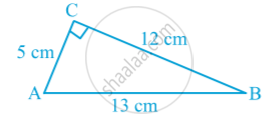Share

# Introduction to Area of a Triangle

#### notes

The triangle is right angled, we can directly apply the formula by using two sides containing the right angle as base and height.

 Area of a triangle = 1/2 xx base xx height

For example :
suppose that the sides of a right triangle ABC are 5 cm, 12 cm and 13 cm; we take base as 12 cm and height as 5 cm in following fig.Then the area of ∆ ABC is given by
1/2 xx base xx height
= 1/2 xx 12 xx 5  cm^2,  i.e., 30 "cm"^2.

S